# Exponential & Logarithmic Graphs

## Exponential Graphs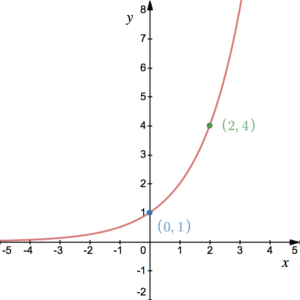Exponential graphs are those of the form $y=a^x$ for positive a. You can sketch the graph of $y=a^x$ for positive a by considering the $y$ coordinates that correspond to various $x$ values. Graphs of this form will always cross the $y$-axis at 1 since $a^0=1$ for any $a$.

The following table shows coordinates for the graph $y=2^x$ for $x$ taking integer values between -3 and 3:

 x -3 -2 -1 0 1 2 3 y 0.125 0.25 0.5 1 2 4 8

It follows that the graph of $y=a^x$ for $a>1$ will have a shape like $y=2^x$.

The graph of $y=a^x$ for $0< a< 1$ will have a shape like the graph above but will be reflected in the $y$-axis. This is because when you multiply a number less than 1 by itself, it becomes smaller.

The following table shows coordinates for the graph $y=0.5^x$ for $x$ taking integer values between -3 and 3:

 x -3 -2 -1 0 1 2 3 y 8 4 2 1 0.5 0.25 0.125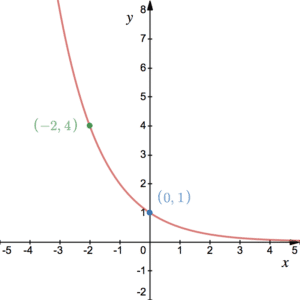For any number $0<a<1$, the graph will have the same shape as $y=0.5^x$.

For $a=1$, the graph of $y=a^x$ is the horizontal line $y=1$. This is because you are calculating 1 to any power, which is always 1. For negative a, fractional powers become an issue and complex numbers need to be considered.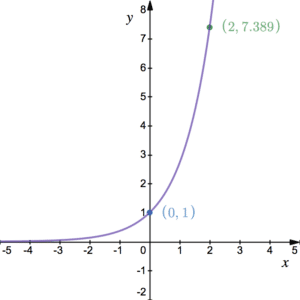The diagram shows the graph of $y=e^{x}$ where $e$, sometimes known as Euler’s number, is given by $e=2.718281828459$… Since $e$ is positive and greater than 1, it looks very similar to the first graph above.

The number $e$ is special because everywhere on this graph, the gradient is the same as the $y$-coordinate. See differentiating $e$ to the $x$.

## Logarithmic Graphs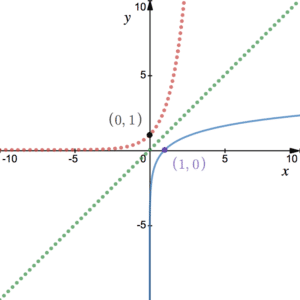As well as exponential graphs, there are logarithmic graphs. $\log_a(x)$ is considered to be the inverse of $a^x$ – see more on logs. It follows that $\log_a(x)$ (blue solid line) is the inverse of $a^x$ (red dotted line) and so their graphs are reflections of each other in the line $y=x$ (green dotted line). Since $\log_a(x)$ and $a^x$ are mathematical inverses we have that $\log_a\left(a^x\right)=a^{\log_a(x)}=x$.
Specifically, the natural logarithm is the logarithm that corresponds to $e$. That is, given an equation of the form $y=e^{x}$, it can be said that $x=\log_e(y)$. Since $e$ is a special number, log to the base $e$ has its own name. That is, it is the natural logarithm and often called $\ln$ so $x=\log_e(y)$ is more often written as $x=\ln(y)$.

$\ln(x)$ and $e^x$ are mathematical inverses and we have that $\ln\left(e^x\right)=e^{\ln(x)}=x$. Notice that $\log_a$ for any positive $a$ (including $\ln$) cannot be evaluated for negative $x$ – see more on logs.

## Estimating Parameters

### Estimating Parameters for $y=ax^n$

Consider the equation $y=ax^n$. Note that, according to BIDMAS, this is $x$ to the power of $n$, then multiplied by $a$. This is a stretch to a standard polynomial curve – see Curve Sketching. Given this relationship and a dataset that approximately fits it, it is possible to estimate the parameters $a$ and $n$. First consider what happens when logging both sides: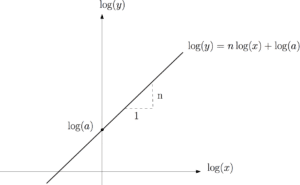$\begin{array}{c}\log(y)=\log(ax^n)\\ \Longrightarrow \log(y)=\log(a)+\log(x^n)\\ \Longrightarrow \log(y)=\log(a)+n\log(x)\end{array}$

Note that the bases are missing this is true for any base (provided the same base is used for both). In the same way that you can plot y against $x$, it is possible to plot $\log(y)$ against $\log(x)$. Recall that, in the equation $y=mx+c$, $m$ is the gradient and $c$ is the $y$-intercept. In addition, we can write $\log(y)$ as $n\log(x)+\log(a)$ and so, in the plot of $\log(y)$ against $\log(x)$, $n$ is the gradient and $\log(a)$ is the $y$-intercept.

### Estimating Parameters for $y=kb^x$

Now consider the equation $y=kb^x$. Like the above, this is an exponential curve, provided $b$ is positive. Similarly to before, given a dataset or similar, we could estimate the parameters $b$ and $k$. Taking logs: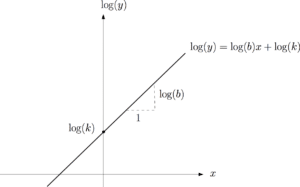$\begin{array}{l}\log(y)=\log(kb^x)\\ \Longrightarrow \log(y)=\log(k)+\log(b^x)\\ \Longrightarrow \log(y)=\log(k)+x\log(b)\end{array}$

It follows that $\log(y)$ can be written as $\log(b)x+\log(k)$ and so, this time, in the plot of $\log(y)$ against $x$, $\log(b)$ is the gradient and $\log(k)$ is the $y$-intercept.

## Examples of Logarithmic Graphs

The following table follows the relationship $y=ax^n$ where the $y$ values are given to one decimal place. By plotting $\log(y)$ against $\log(x)$, this allows us to estimate the parameters a and $n$ to 1 decimal place.

 x 2 3 4 5 y 26.4 104.7 278.6 594.9